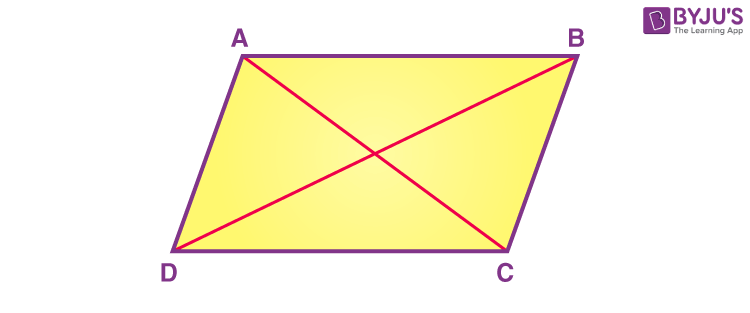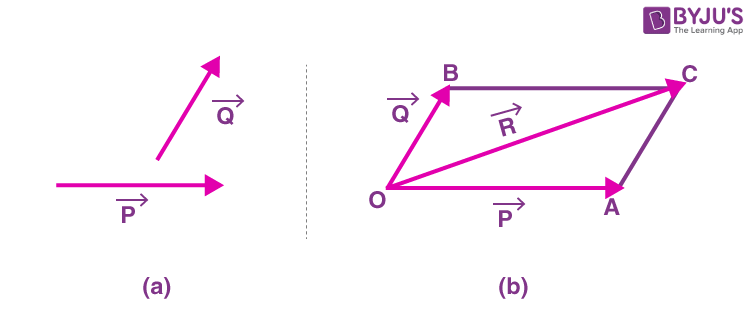# Parallelogram Law

In Mathematics, the parallelogram law is the fundamental law that belongs to elementary Geometry. This law is also known as parallelogram identity. In this article, let us look at the definition of a parallelogram law, proof, and parallelogram law of vectors in detail.

The Parallelogram law states that the sum of the squares of the length of the four sides of a parallelogram is equal to the sum of the squares of the length of the two diagonals. In Euclidean geometry, it is necessary that the parallelogram should have equal opposite sides.If ABCD is a parallelogram, then AB = DC and AD = BC. Then according to the definition of the parallelogram law, it is stated as

2(AB)2 + 2 (BC)2 = (AC)2 + (BD)2.

In case the parallelogram is a rectangle, then the law is stated as:

2(AB)2 + 2 (BC)2 = 2(AC)2

This is because in a rectangle, two diagonals are of equal lengths. i.e., (AC = BD)

## Parallelogram Law of VectorsIf two vectors are acting simultaneously at a point, then it can be represented both in magnitude and direction by the adjacent sides drawn from a point. Therefore, the resultant vector is completely represented both in direction and magnitude by the diagonal of the parallelogram passing through the point.

Consider the above figure,

The vector P and vector Q represents the sides, OA and OB, respectively.

According to the parallelogram law, the side OC of the parallelogram represents the resultant vector R.

$$\begin{array}{l}\vec{OA}+\vec{OB}= \vec{OC}\end{array}$$
(or)

$$\begin{array}{l}\vec{P}+\vec{Q}= \vec{R}\end{array}$$

### Parallelogram Law of Addition of Vectors Procedure

The steps for the parallelogram law of addition of vectors are:

• Draw a vector using a suitable scale in the direction of the vector
• Draw the second vector using the same scale from the tail of the first vector
• Treat these vectors as the adjacent sides and complete the parallelogram
• Now, the diagonal represents the resultant vector in both magnitude and direction

### Parallelogram Law Proof

Let AD=BC = x, AB = DC = y, and ∠ BAD = α

Using the law of cosines in the triangle BAD, we get

x2 + y2 – 2xy cos(α) = BD2 ——-(1)

We know that in a parallelogram, the adjacent angles are supplementary. So

Now, again use the law of cosines in the triangle ADC

x2 + y2 – 2xy cos(180 – α) = AC2 ——-(2)

Apply trigonometric identity cos(180 – x) = – cos x in (2)

x2 + y2 + 2xy cos(α) = AC2

Now, the sum of the squares of the diagonals (BD2 + AC2) are represented as,

BD2 + AC2  = x2 + y2 – 2xycos(α) + x2 + y2 + 2xy cos(α)

Simplify the above expression, we get;

BD2 + AC2 =2x2 + 2 y2 ——-(3)

The above equation is represented as:

BD2 + AC2 = 2(AB)2 + 2(BC)2

Hence, the parallelogram law is proved.

Stay tuned with BYJU’S – The Learning App for Maths concepts, theorems, proof and examples.

 Related Links Parallelogram Rectangle Law of Cosines Trigonometry